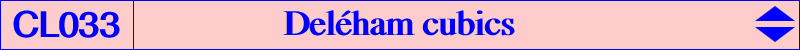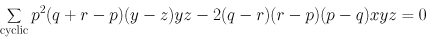This page was written from an idea by Philippe Deléham and with his cooperation. Consider a fixed point P = p : q : r and a variable point M. Denote by A', B', C' the midpoints of ABC. The lines AM and PA' meet at Ma. Mb and Mc are defined similarly. These points Ma, Mb, Mc are collinear on a line L if and only if M lies on the Deléham cubic Del(P). Del(P) is therefore a special case of Grassmann cubic. Here, the line L envelopes the inscribed parabola (P) whose perspector S = 1 / [(q-r)(q+r-p)] : : is the isotomic conjugate of W, the infinite point of the trilinear polar of Q = anticomplement of P. Thus, S lies on the Steiner ellipse. The focus of (P) is naturally F, the isogonal conjugate of W. The trilinear polar Ls of S passes through G and the isotomic conjugate of the anticomplement of P. An easy (with ruler only) construction of Del(P) is the following : for any point N on Ls, the trilinear polar of N meets PA', PB', PC' at Na, Nb, Nc. The lines ANa, BNb, CNc concur on Del(P). The general equation of Del(P) is :Del(P) is psK(P x G/P, G, P) where G/P is the center of the circum-conic with perspector P. Properties of Del(P) : Del(P) is a nodal circum-cubic with node P and contains : the midpoints A', B', C', Q = aP = anticomplement of P, P1 = G-Ceva conjugate of P^2 = p^2(q^2+r^2-p^2) : : , P2 = (G-Ceva conjugate of P)^2 = p^2(q+r-p)^2 : : , P3 = 1 / [q+r-p)^2 - 2qr] : : = cross-conjugate of Q' and T, with Q' = isotomic conjugate of Q = 1 / (q+r-p) : : and T = p^2(q+r-p) : : . It decomposes into the medians when P = G. When P = O, we find the Lemoine cubic K009 and when P = X(9), we find the Mittencubic K220. See also K259 and K260. The nodal tangents at P are perpendicular if and only if P lies on the Thomson cubic K002. This is the case for the Lemoine cubic K009. These nodal tangents are parallel to the asymptotes of the rectangular hyperbola passing through P. The tangents at A, B, C are concurrent at the point T = p^2(q+r-p) : : . and those at A', B', C' concur if and only if P lies on the anticomplement of K016, the Tucker+ cubic. Del(P) contains O if and only if P lies on K258. In this case, there is an isogonal pK having the same asymptotic directions and another isogonal pK having the same common points with the circumcircle. With P = O, these cubics are the Orthocubic K006 and the McCay cubic K003 respectively. With P = X(1), they are pK(X6, X145) and pK(X6, X8) and the corresponding Deléham cubic is K259. The following table gives a selection of points P such that S, W, F are clearly identified. Ds is the directrix of (P) i.e. the Steiner line of F. Remember that Ls is the trilinear polar of S. Remark that Ds passes through P if and only if P lies on the Thomson cubic. Conversely, if the line Ls (through G) is given, P must lie on its G-Ceva conjugate which is a conic passing through G and the midpoints A', B', C'. This conic is in fact the complement of the circum-conic whose perspector is the infinite point of Ls. It is a rectangular hyperbola if and only if Ls is the line GK and then it also contains X(3), X(39), X(114), X(618), X(619), X(629), X(630), X(641), X(642), X(1125), X(1649). It is the complement of the Kiepert hyperbola.P Del(P) S W F Ds Ls remarks / other points on Del(P) X(1) K259 X(664) X(522) X(109) X(4)-X(1) X(2)-X(7) X(3) K009 X(99) X(523) X(110) Euler line X(2)-X(6) (P) is the Kiepert parabola X(6) K260 X(648) X(525) X(112) X(4)-X(6) Euler line X(9) K220 X(190) X(514) X(101) X(4)-X(9) X(2)-X(1) (P) is the Yff parabola X(10) X(668) X(513) X(100) X(4)-X(8) X(2)-X(37) X(1), X(12), X(1500) X(115) X(671) X(524) X(111) X(4)-X(1499) X(2)-X(523) X(99), X(339) X(125) X(290) X(511) X(98) X(4)-X(512) X(2)-X(647) X(110), X(136) X(126) X(670) X(512) X(99) X(4)-X(69) X(2)-X(39) X(111), X(1560) X(512) X(886) X(888) X(888)* X(4)-? X(2)-X(670) X(513) X(889) X(891) X(898) X(4)-? X(2)-X(668) X(798) X(523) X(892) X(690) X(691) X(4)-X(542) X(2)-X(99) X(650) X(666) X(918) X(919) X(4)-X(218) X(2)-X(11) X(661), X(693) X(1086) X(903) X(519) X(106) X(4)-? X(2)-X(514) X(190) X(1146) X(1121) X(527) X(2291) X(4)-? X(2)-X(522) X(664), X(1111) X(1650) X(1494) X(30) X(74) X(4)-X(523) X(2)-X(525)